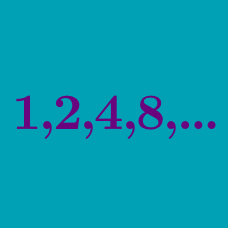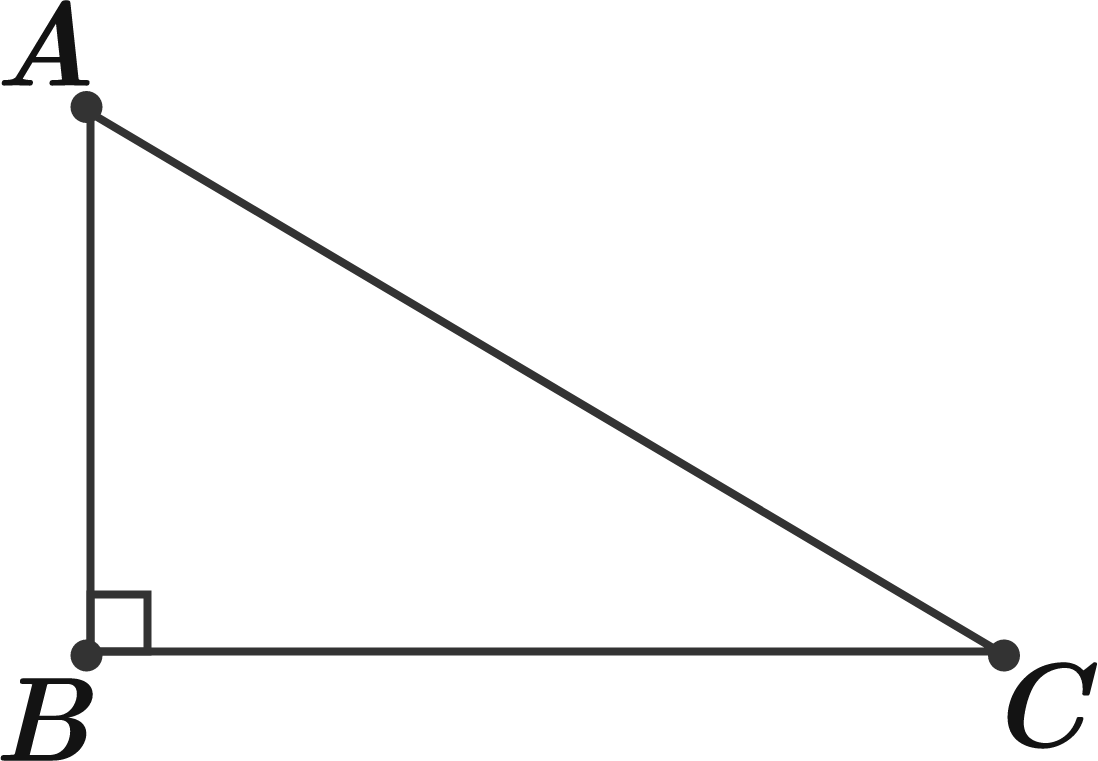Algebra

# Geometric Progressions: Level 3 Challenges

If the lengths of sides $$AB,$$ $$BC$$ and $$AC$$ in the figure shown form a geometric progression in that order, what is the ratio between $$AC$$ and $$AB$$ to 3 decimal places?$(\log_{b} 2)^{0}\left(\log_{b} 5^{2^{0}}\right) + (\log_{b} 2)^{1}\left(\log_{b} 5^{2^{1}}\right) + (\log_{b} 2)^{2}\left(\log_{b} 5^{2^{2}}\right) + \cdots$

If $$b = 100,$$ what is the infinite sum above?

$\{ a + \log_2 3, a + \log_4 3, a + \log_8 3 \}$

Let $$a$$ be a real number such that the above set of numbers form a geometric progression (in that order). Find the common ratio of this geometric progression.

$\Large \sqrt {2}\times \sqrt{4}\times \sqrt{8}\times \sqrt{16}\times \cdots = \ ?$

$1= x^{2} - x^{3} + x^{4} -x^{5} +\ldots$Solve for $$x$$ in the equation above.
If the sum of all values of $$x$$ can be represented in the form $$\dfrac{a+b\sqrt{c}}{d}$$, such that $$a,b,c$$ and $$d$$ are integers and the fraction is in lowest form and $$d> 0$$, find $$a+b+c+d$$.